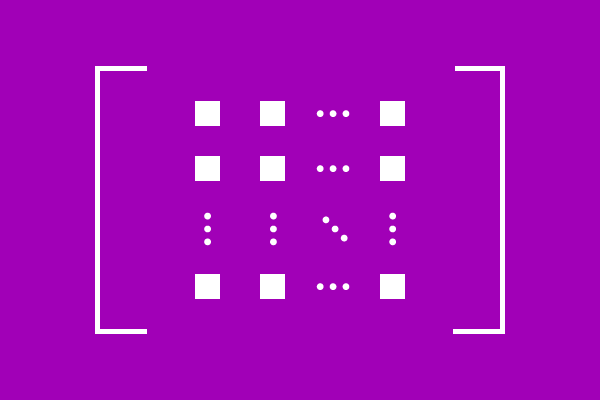# Square Matrix

## Definition

A matrix whose number of rows equals to the number of columns, is called a square matrix.Square matrix is one type of matrix and elements are arranged in number of rows and columns but the arrangement of elements in matrix is in square shape due to same number of rows and columns. Hence, the matrix is called as a square matrix.

A square matrix is expressed in general form as follows. In this matrix, the elements are arranged in $m$ rows and $n$ columns and the order of matrix is $m \times n$.

$$M = {\begin{bmatrix} e_{11} & e_{12} & e_{13} & \cdots & e_{1n}\\ e_{21} & e_{22} & e_{23} & \cdots & e_{2n}\\ e_{31} & e_{32} & e_{33} & \cdots & e_{3n}\\ \vdots & \vdots & \vdots & \ddots & \vdots \\ e_{m1} & e_{m2} & e_{m3} & \cdots & e_{mn} \end{bmatrix}}_m \times n}$$

Square shape in matrix is possible when the number of rows is equal to number of columns, which means $m = n$. Therefore, if any matrix having same number of rows and columns, then the matrix is known a square matrix.

A square matrix is usually called in three different ways according to order of the matrix.

1. A square matrix of order $m$ or $n$.
2. $m^{th}$ or $n^{th}$ order square matrix.
3. $m$ or $n$ rowed square matrix.

### Example

$$M = \begin{bmatrix} 7 & -2 & 5 & 4\\ -1 & 0 & 3 & -7\\ 9 & 4 & 2 & 5\\ -5 & 3 & 8 & 4 \end{bmatrix}$$

$M$ is an example matrix. It has $4$ rows and $4$ columns. The matrix $M$ is called a square matrix. The $4 \times 4$ matrix is also called in three different types.

1. A square matrix of order $4$.
2. Fourth order square matrix.
3. $4$ rowed square matrix.

Observe the following few more examples.

$$A = \begin{bmatrix} 6 \end{bmatrix}$$

$A$ is a matrix of order $1 \times 1$. It is called as a square matrix of order $1$ or first order square matrix or $1$ rowed square matrix.

$$B = \begin{bmatrix} 1 & 5\\ 3 & 4 \end{bmatrix}$$

$B$ is a matrix of order $2 \times 2$. It is called as a square matrix of order $2$ or second order square matrix or $2$ rowed square matrix.

$$C = \begin{bmatrix} 8 & 2 & 5\\ 0 & 3 & 6\\ 4 & -2 & 9 \end{bmatrix}$$

$C$ is a matrix of order $3 \times 3$. It is called as a square matrix of order $3$ or third order square matrix or $3$ rowed square matrix.

Latest Math Topics
Jun 26, 2023
Jun 23, 2023

###### Math Questions

The math problems with solutions to learn how to solve a problem.

Learn solutions

Practice now

###### Math Videos

The math videos tutorials with visual graphics to learn every concept.

Watch now

###### Subscribe us

Get the latest math updates from the Math Doubts by subscribing us.